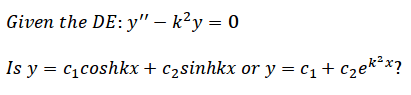# Which solution for this DE is preferred?

TurionAre they both correct? Would the first or second solution be preferred?

The second one is certainly not a solution. There is a solution in terms of exponentials, but that is not it.

Turion
The second one is certainly not a solution. There is a solution in terms of exponentials, but that is not it.

Why is the second one not a solution? If you convert the original DE into an auxiliary equation, you will get roots: m1=0 and m2=k2

Staff Emeritus
Gold Member
The first of your solutions can be arrived at from the second. I would say that the second is the fundamental.

Why is the second one not a solution? If you convert the original DE into an auxiliary equation, you will get roots: m1=0 and m2=k2

No you don't. You get roots of +k and -k. Try plugging your second solution into the DE and see if it works. You'll see that it doesn't.

Turion
No you don't. You get roots of +k and -k. Try plugging your second solution into the DE and see if it works. You'll see that it doesn't.

That's weird: http://www.wolframalpha.com/input/?i=m^2-k^2m=0

Going to try plugging it in.

Edit: Oh wow. You're right. I'm an idiot. Lol

The characteristic equation for the D.E. you give, $y''- k^2y= 0$ is $r^2- k= 0$ which is equivalent to $r^2= k^2$ and has roots k and -k. You, apparently, miswrote the equation as $y''- k^2y'= 0$, which has characteristic equation $r^2- k^2r= r(r- k^2)= 0$ and has roots 0 and $k^2$.